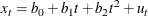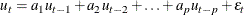### Combining Time Trend with Autoregressive Models

Trend models are suitable for capturing long-term behavior, whereas autoregressive models are more appropriate for capturing short-term fluctuations. One approach to forecasting is to combine a deterministic time trend model with an autoregressive model.

The stepwise autoregressive method (STEPAR method) combines a time trend regression with an autoregressive model for departures from trend. The combined time trend and autoregressive model is written as follows:The autoregressive parameters included in the model for each series are selected by a stepwise regression procedure, so that autoregressive parameters are included only at those lags at which they are statistically significant.

The stepwise autoregressive method is fully automatic. Unlike the exponential smoothing and Winters methods, it does not depend on choosing smoothing weights. However, the STEPAR method assumes that the long-term trend is stable; that is, the time trend regression is fit to the whole series with equal weights for the observations.

The stepwise autoregressive model is used when you specify the METHOD=STEPAR option or do not specify any METHOD= option. To select a constant, linear, or quadratic trend for the time-trend part of the model, use the TREND= option.